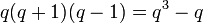# Linear representation theory of special linear group of degree two over a finite field

## Contents

This article gives specific information, namely, linear representation theory, about a family of groups, namely: special linear group of degree two.
View linear representation theory of group families | View other specific information about special linear group of degree two

This article describes the linear representation theory of the special linear group of degree two over a finite field. The order (size) of the field is$q$, and the characteristic prime is$p$.$q$ is a power of$p$. We denote the group as$SL(2,q)$ or$SL_2(q)$.

See also the linear representation theories of: general linear group of degree two, projective general linear group of degree two, and projective special linear group of degree two.

For linear representation theory in characteristics that divide the order of the group, refer:

• Modular representation theory of special linear group of degree two over a finite field in its defining characteristic: In characteristic$p$, same as the field characteristic.
• (Modular representation theory in other characteristics that divide$q - 1$ or$q + 1$ -- link to be added)

## Summary

Item Value
degrees of irreducible representations over a splitting field (such as$\overline{\mathbb{Q}}$ or$\mathbb{C}$) Case$q$ odd: 1 (1 time),$(q - 1)/2$ (2 times),$(q + 1)/2$ (2 times),$q - 1$ ($(q - 1)/2$ times),$q$ (1 time),$q + 1$ ($(q - 3)/2$ times)
Case$q$ even: 1 (1 time),$q - 1$ ($q/2$ times),$q$ (1 time),$q + 1$ ($(q - 2)/2$ times)
number of irreducible representations Case$q$ odd:$q + 4$; Case$q$ even:$q + 1$.
See number of irreducible representations equals number of conjugacy classes, element structure of special linear group of degree two over a finite field#Conjugacy class structure
quasirandom degree (minimum degree of nontrivial irreducible representation) Case$q$ odd:$(q - 1)/2$
Case$q$ even:$q - 1$
maximum degree of irreducible representation over a splitting field$q + 1$ if$q>3$$q$ if$q \in \{2,3\}$
lcm of degrees of irreducible representations over a splitting field Case$q = 3$: We get 6
Case$q$ odd,$q > 3$:$q(q+1)(q-1)/2 = (q^3-q)/2$
Case$q$ even:$q(q+1)(q-1) = q^3 - q$
sum of squares of degrees of irreducible representations over a splitting field$q^3 - q$, equal to the group order. See sum of squares of degrees of irreducible representations equals group order

## Particular cases$q$ (field size)$p$ (underlying prime, field characteristic) Case for$q$ Group$SL(2,q)$ Order of the group ($q^3 - q$) Degrees of irreducible representations (ascending order) Number of irreducible representations ($q + 4$ if$q$ odd,$q + 1$ if$q$ even) Linear representation theory page
2 2 even symmetric group:S3 6 1,1,2 3 linear representation theory of symmetric group:S3
3 3 odd special linear group:SL(2,3) 24 1,1,1,2,2,2,3 7 linear representation theory of special linear group:SL(2,3)
4 2 even alternating group:A5 60 1,3,3,4,5 5 linear representation theory of alternating group:A5
5 5 odd special linear group:SL(2,5) 120 1,2,2,3,3,4,4,5,6 9 linear representation theory of special linear group:SL(2,5)
7 7 odd special linear group:SL(2,7) 336 1,3,3,4,4,6,6,6,7,8,8 11 linear representation theory of special linear group:SL(2,7)
8 2 even projective special linear group:PSL(2,8) 504 1,7,7,7,7,8,9,9,9 9 linear representation theory of projective special linear group:PSL(2,8)
9 3 odd special linear group:SL(2,9) 720 1,4,4,5,5,8,8,8,8,9,10,10,10 13 linear representation theory of special linear group:SL(2,9)